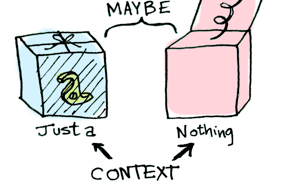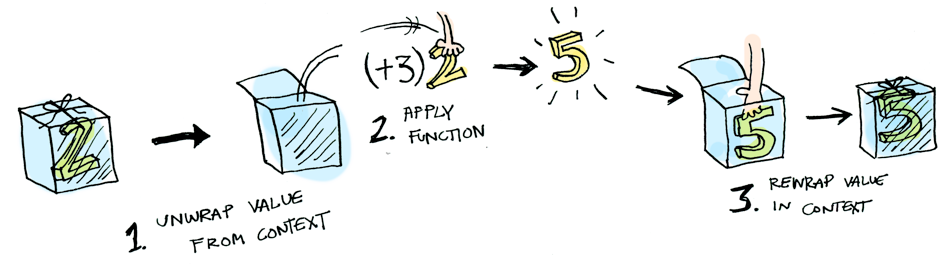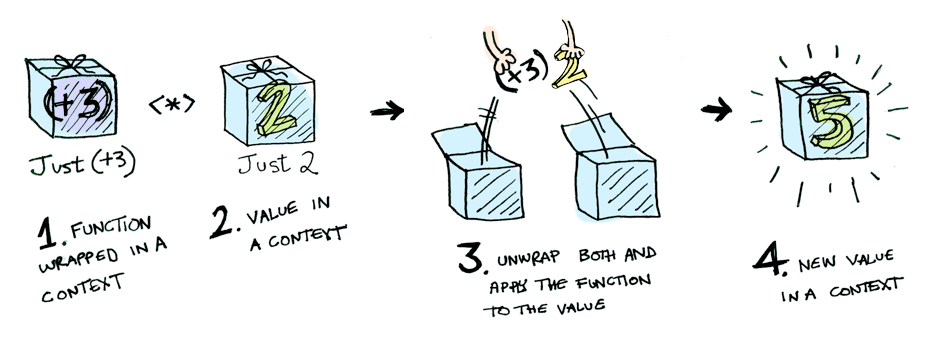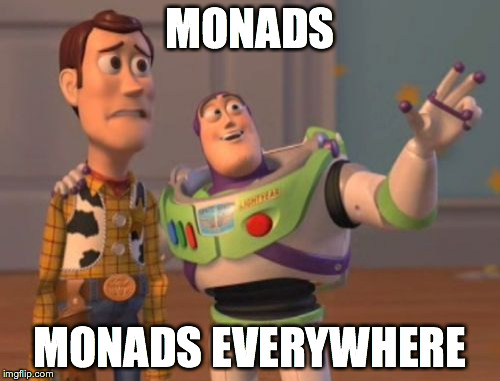# Preface

This post has many awesome pictures which credits go to Aditya Bhargava. His original article Functors, Applicatives, And Monads In Pictures is extremely well written, with sample code in Haskell though.

In this post I will try to provide proof of concept of Functor/Applicatives/Monad in pure Swift, plus example for using Reader Monad for Dependency Injection(DI), and the idea of Try monad concept from Scala.

# Maybe is Functor

We all know the Optional Type (the ? mark) in Swift. We can define our option type named `Maybe` using enum.

```enum Maybe<T> {
case Just(T)
case Nothing
}
```

Simple enough! A `Maybe` type is a "box" which can contains the value or ... nothingThe interesting part come from here: we can define a `fmap` function which takes a normal function and a `Maybe` type, then return another `Maybe`How does `fmap` look like? Well, its implement is not that hard

```extension Maybe {
func fmap<U>(f: T -> U) -> Maybe<U> {
switch self {
case .Just(let x): return .Just(f(x))
case .Nothing: return .Nothing
}
}
}
```

And the "magic" above is actually not-so-magical. At this time, our `Maybe` type is already a Functor.# Maybe is Applicatives

Applicatives is a type which can define the function `apply` that

• Take a function wrapped in that type
• Take also a value wrapped in that type
• Then return a new value which is wrapped alsoI will define an `apply` function for `Maybe`

```extension Maybe {
func apply<U>(f: Maybe<T -> U>) -> Maybe<U> {
switch f {
case .Just(let JustF): return self.fmap(JustF)
case .Nothing: return .Nothing
}
}
}
```

That is it! Our `Maybe` now is both Functor and Applicatives.

# Maybe is Monad

How to learn about Monads:

1. Get a PhD in computer science.
2. Throw it away because you don’t need it for this section

`Maybe` can be considered as a monad if it can define a function `flatmap` that

• Take a function which return type is `Maybe`
• Take also a value wrapped in `Maybe`
• Return another `Maybe`

Let's Swift! Here is our `flatMap` and bind operator `>>=`

```extension Maybe {
func flatMap<U>(f: T -> Maybe<U>) -> Maybe<U> {
switch self {
case .Just(let x): return (f(x))
case .Nothing: return .Nothing
}
}
}
infix operator >>= { associativity left }
func >>=<T, U>(a: Maybe<T>, f: T -> Maybe<U>) -> Maybe<U> {
return a.flatMap(f)
}
```

Suppose that we already have a `half` function that return an `Maybe` type

```func half(a: Int) -> Maybe<Int> {
return a % 2 == 0 ? Maybe.Just(a / 2) : Maybe.Nothing
}
```

Then with the `>>=` operator you can chain `Maybe` like:

```Maybe.Just(20) >>= half >>= half >>= half
```

And this is how it is actually processedNow our `Maybe` is Functor, Applicatives and also Monad as well.

# A step further, the Reader monad

In this section I will introduce minimal version for one of three useful Monads: the Reader Monad

```class Reader<E, A> {
let g: E -> A
init(g: E -> A) {
self.g = g
}
func apply(e: E) -> A {
return g(e)
}
func map<B>(f: A -> B) -> Reader<E, B> {
return Reader<E, B>{ e in f(self.g(e)) }
}
func flatMap<B>(f: A -> Reader<E, B>) -> Reader<E, B> {
return Reader<E, B>{ e in f(self.g(e)).g(e) }
}
}
```As you can see, we have `map`, and `flatMap` function here. This class type is both Functor and Monad at the same time. Very same with `Maybe` monad above, `Reader` can define infix operator and chain to whenever we want.

```infix operator >>= { associativity left }
func >>=<E, A, B>(a: Reader<E, A>, f: A -> Reader<E, B>) -> Reader<E, B> {
return a.flatMap(f)
}

func half(i: Float ) -> Reader<Float , Float> {
return Reader{_ in i/2}
}
let f = Reader{i in i} >>= half >>= half >>= half
f.apply(20) // 2.5
```

## Why Reader monad matter

Reader monad take `g` function in `init` time. By switching (or injecting) this function, we can create our own Dependency Injection(DI) framework easily. Let's see an example:

This is our model:

```struct User {
var name: String
var age: Int
}
struct DB {
var path: String
func findUser(userName: String) -> User {
// DB Select operation
return User(name: userName, age: 29)
}
func updateUser(u: User) -> Void {
// DB Update operation
print(u.name + " in: " + path)
}
}
```

and usage:

```let dbPath = "path_to_db"
func update(userName: String, newName: String) -> Void {
let db = DB(path: dbPath)
var user = db.findUser(userName)
user.name = newName
db.updateUser(user)
}
update("dummy_id", newName: "Thor")
// Thor in: path_to_db
```

In real life `DB` may be compicated and seperated as a whole infrastructure layer. Assume that `DB` can find an user by his name and update his information to the Database.

The problem is `update` function now holding a reference to `dbPath`, which I want to switch during test or runtime. I will rewrite the `update` function to return only a `Reader`

```struct Environment {
var path: String
}
func updateF(userName: String, newName: String) -> Reader<Environment, Void> {
return Reader<Environment, Void>{ env in
let db = DB(path: env.path)
var user = db.findUser(userName)
user.name = newName
db.updateUser(user)
}
}
```

then call `Reader.apply` later base on what passed through Environment variable.

```let test = Environment(path: "path_to_sqlite")
let production = Environment(path: "path_to_realm")
updateF("dummy_id", newName: "Thor").apply(test)
// Thor in: path_to_sqlite
updateF("dummy_id", newName: "Thor").apply(production)
// Thor in: path_to_realm
```# The Try Monad

Scala's `Try` type is a functional approach for error handling. Very likely to Optional (or `Maybe`), `Try` is a "box" that contains value or a Throwable if something has gone wrong. `Try` can be a Successful or a Failure.

```enum Try<T> {
case Successful(T)
case Failure(ErrorType)
init(f: () throws -> T) {
do {
self = .Successful(try f())
} catch {
self = .Failure(error)
}
}
}
```

To make `Try` a functor/monad, I will add `map` and `flatMap` function

```extension Try {
func map<U>(f: T -> U) -> Try<U> {
switch self {
case .Successful(let value): return .Successful(f(value))
case .Failure(let error): return .Failure(error)
}
}
func flatMap<U>(f: T -> Try<U>) -> Try<U> {
switch self {
case .Successful(let value): return f(value)
case .Failure(let error): return .Failure(error)
}
}
}
```

With an operation which can throws some ErrorType, just wrap them inside a Try and chain(with `map` and `flatMap`) to whenever you want. At every step the result will be a `Try` type. When you want the real value inside that box, just do a pattern matching.

```enum DoomsdayComing: ErrorType {
case Boom
case Bang
}
let endOfTheWorld = Try {
throw DoomsdayComing.Bang
}
let result = Try {4/2}.flatMap { _ in endOfTheWorld}
switch result {
case .Successful(let value): print(value)
case .Failure(let error): print(error)
}
// Bang
```

# Conclusion

1. A functor is a type that implements map.
2. An applicative is a type that implements apply.
3. A monad is a type that implements flatMap.• `Maybe` have map, apply and flatMap, so it is a functor, an applicative, and a monad.
• `Reader` is a monad which can be used for DI(Dependency Injection)
• `Try` is a monad, and so as `Future`, `Signal` or `Observable` (see their `flatMap` implement!)Thanks for reading this article and feel free to give any feedback or suggestion. You can open a pull request or reach me out at @dtvd88. If you want to play around with above class, clone this repo and open the Playground.

Many thanks to Aditya Bhargava for his awesome blog.

Get A Weekly Email With Trending Projects For These Topics
No Spam. Unsubscribe easily at any time.
swift (7,627
monads (25
functor (18